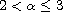Electron. J. Differential Equations, Vol. 2017 (2017), No. 203, pp. 1-15.

### Lyapunov-type inequalities for-th order fractional differential equations withand fractional boundary conditions Sougata Dhar, Qingkai Kong

Abstract:
We study linear fractional boundary value problems consisting of an-th order Riemann-Liouville fractional differential equation withand certain fractional boundary conditions. We derive several Lyapunov-type inequalities and apply them to establish nonexistence, uniqueness, and existence-uniqueness of solutions for related homogeneous and nonhomogeneous linear fractional boundary value problems. As a special case, our work extends some existing results for third-order linear boundary value problems.

Submitted June 2, 2017. Published September 6, 2017.
Math Subject Classifications: 34A08, 34A40, 26A33, 34B05.
Key Words: Fractional differential equations; fractional boundary conditions; Lyapunov-type inequalities; boundary value problems; existence and uniqueness of solutions.

Show me the PDF file (258 KB), TEX file for this article.Sougata Dhar Department of Mathematics and Statistics University of Maine Orono, ME 04469, USA email: sougata.dhar@maine.edu Qingkai Kong Department of Mathematics Northern Illinois University DeKalb, IL 60115, USA email: qkong@niu.edu